# 3D视觉

## 1. 简介

• 从一张 2D 图像预测得到 3D 表示
• 处理 3D 表示数据来实现下游任务（分类、检测、分割等）

## 2. 3D 表示

### 2.1 深度图

#### 2.1.1 RGB-D

• RGB image + Depth image = RGB-D image (2.5D)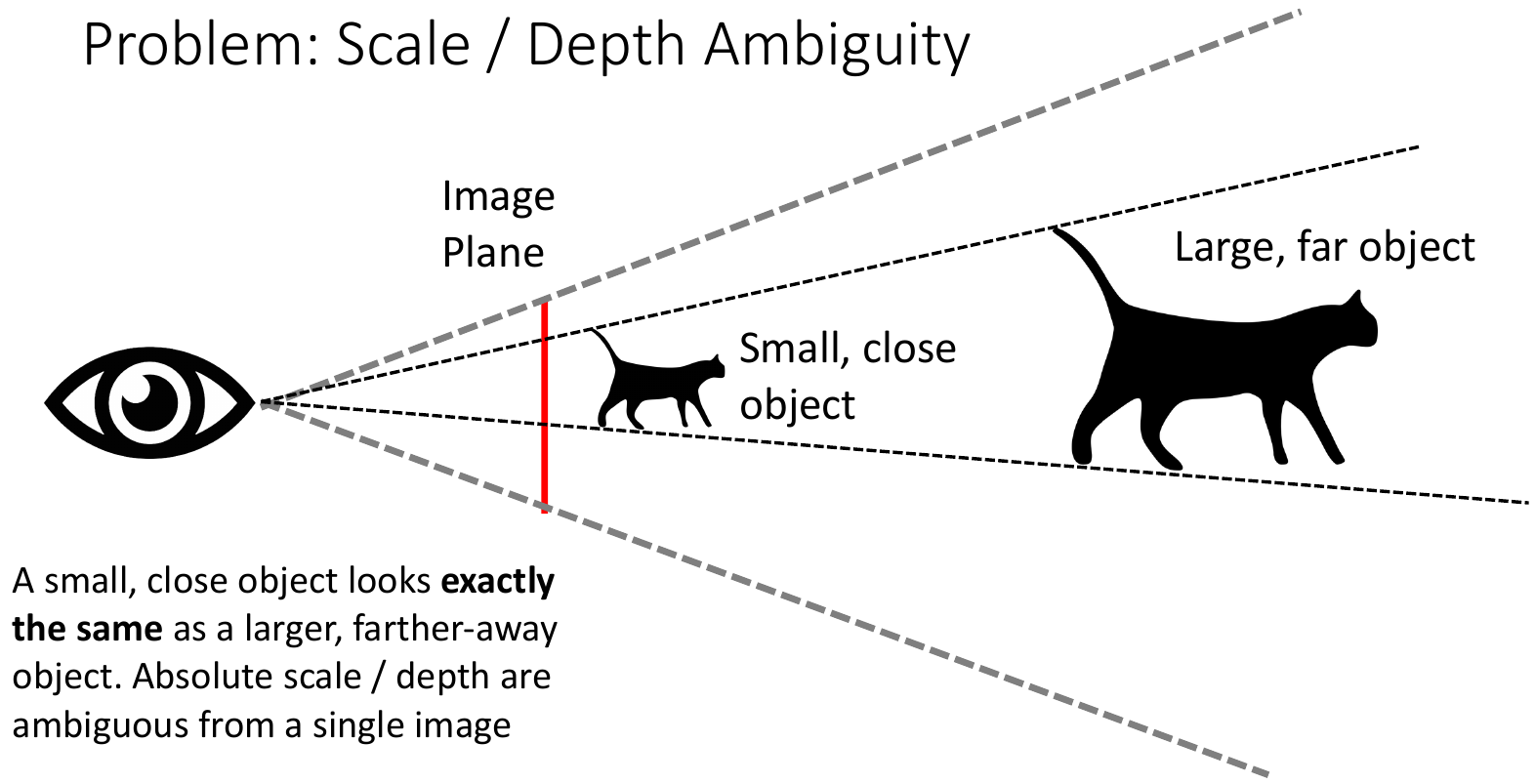\begin{align*} D\left(y, y^{*}\right) & =\frac{1}{n} \sum_{i=1}^{n}\left(\log y_{i}-\log y_{i}^{*}+\alpha\left(y, y^{*}\right)\right)^{2},\text{ 其中 } \alpha\left(y, y^{*}\right)=\frac{1}{n} \sum_{i}\left(\log y_{i}^{*}-\log y_{i}\right) \\ & = \frac{1}{n} \sum_{i=1}^{n}\left(\left(\log y_{i}-\log y_{i}^{*}\right) - \left(\frac{1}{n} \sum_{i}\left(\log y_{i}^{*}-\log y_{i}\right)\right)^2\right)^{2} \\ & = \frac{1}{n} \sum_{i=1}^{n}\left(d_i - \left(\frac{1}{n}\sum_{j=1}^n d_j \right)^2 \right)^2,\text{ 其中 } d_i = \log y_{i}-\log y_{i}^{*} \\ & = \frac{1}{n} \sum_i d_i^2 - \frac{1}{n^2}\left(\sum_j d_j\right)^2 \tag{1} \end{align*}

$y$$y^*$ 的尺度缩放时，即 $y = C y^*$ 时，有 $d = \log{C}$，代入 $(1)$ 可计算得 $D(y, y^*) = 0$。因此，该损失函数即为 scale-invariant 的。

Eigen 等人提出的神经网络结构如下：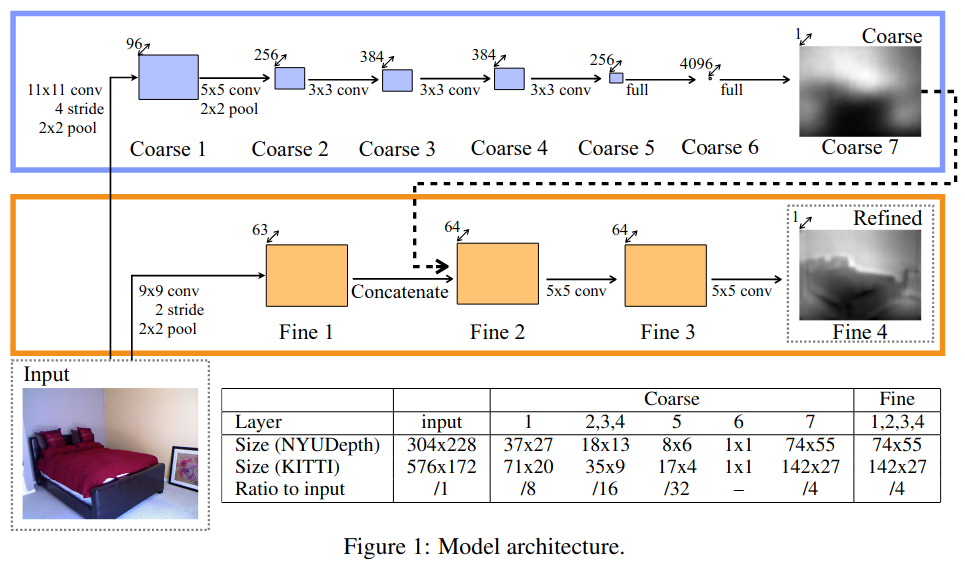#### 2.1.2 RGB + Surface Normal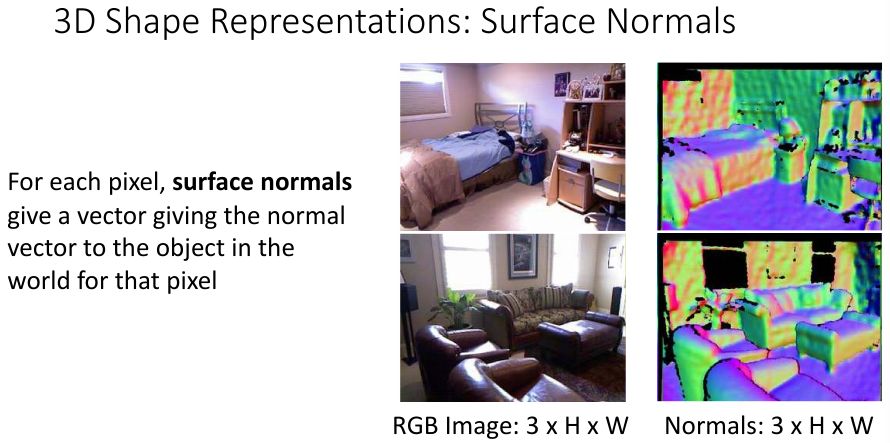Eigen 等人在第二年又提出了从 RGB 图像预测 Depth Map、Surface Normal 和 Semantic Label 的统一神经网络模型，其结构如下图所示：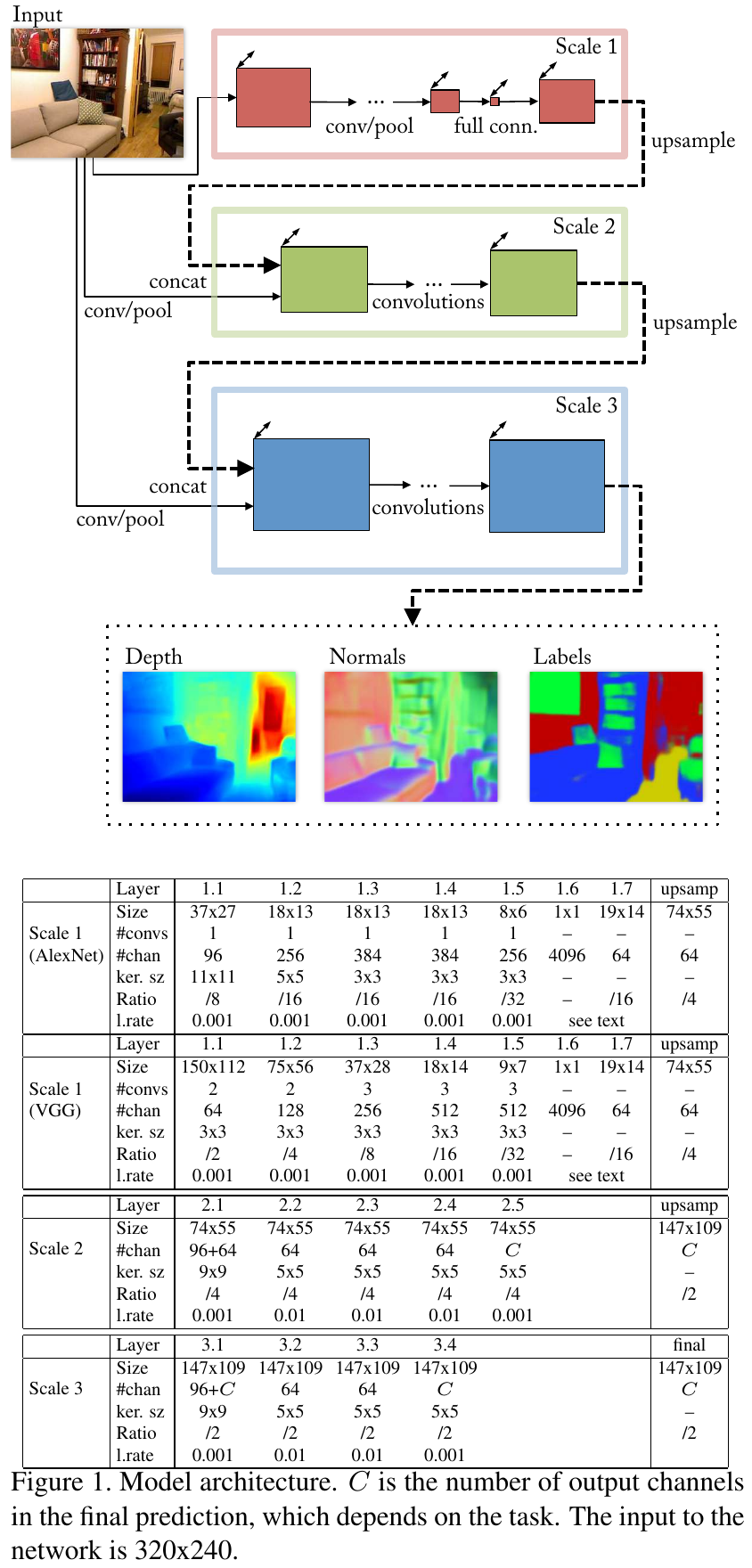• 如果使用该模型来预测 Depth Map，则给出的损失函数为：

\begin{align*} L_{\mathrm{depth}}(D, D^*) = \frac{1}{n} \sum_i d_i^2 - \frac{1}{2n^2} \left(\sum_i d_i\right)^2 + \frac{1}{n} \sum_i \left[ (\nabla_x d_i)^2 + (\nabla_y d_j)^2\right] \tag{2} \end{align*}

其中，$D = \log{y}, D^* = \log{y^*}$$y, y^*$ 分别表示网络预测的深度图和 ground truth。与式 $(1)$ 一样，$d = D - D^*$。相比于式 $(1)$，式 $(2)$ 增加了两个梯度项，$\nabla_x d_i$$\nabla_y d_j$ 分别表示 $d$$x,y$ 轴上的梯度，从而使得 $y$$y^*$ 不仅在数值上相近，在结构上也相近。

不难证明，当 $y$$y^*$ 的尺度缩放时，即 $y = C y^*$ 时，依然有 $L_{\mathrm{depth}}(D, D^*) = 0$，因此该损失也是 scale-invariant 的。

• 如果使用该模型来预测 Surface Normal，则给出的损失函数为：

$L_{\mathrm{normal}}(N, N^*) = -\frac{1}{n} \sum_i N_i \cdot N_i^* \tag{3}$

其中，$N$$N^*$ 分别表示网络预测的 Surface Normal 和 groud truth，$N_i$$N_i^*$ 都是 $3$ 维的单位法向量，$(\cdot)$ 为向量间的点积。

两个单位法向量之间的点积其实就是二都的角度余弦，当二者重合时值最大，当二者反向时值最小，故损失函数加了一个负号。

• 如果使用该模型来预测 Semantic Label，则给出的损失函数为：

$L_{\mathrm{semantic}}(C, C^*) = -\frac{1}{n} \sum_i C_i^* \log{C_i} \tag{4}$

其中，$C_i$ 是网络最后一层经过 Softmax 给出的预测标签，$C_i^*$ 为 ground truth。

### 2.2 体素

#### 2.2.1 体素网络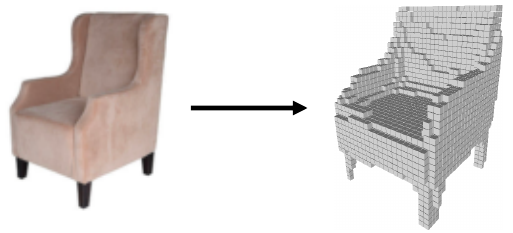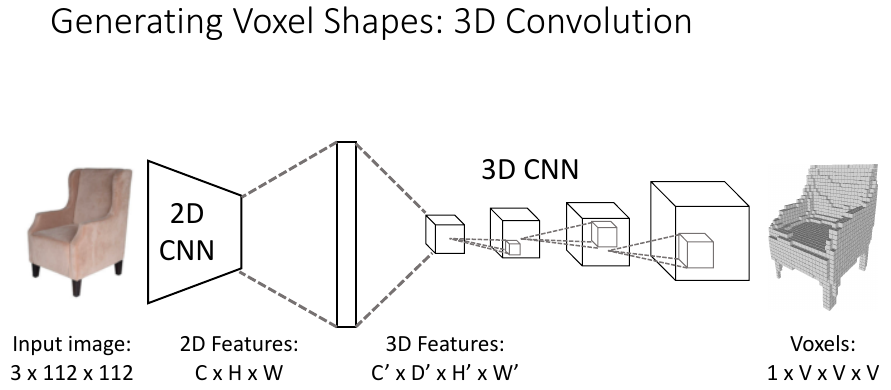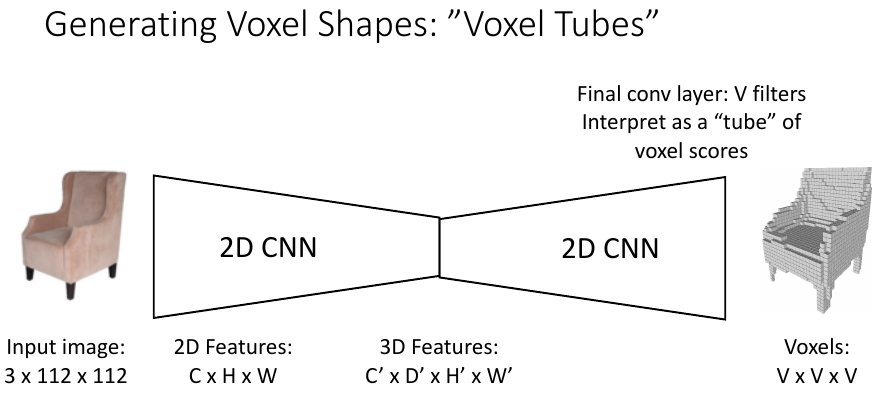#### 2.2.2 多尺度体素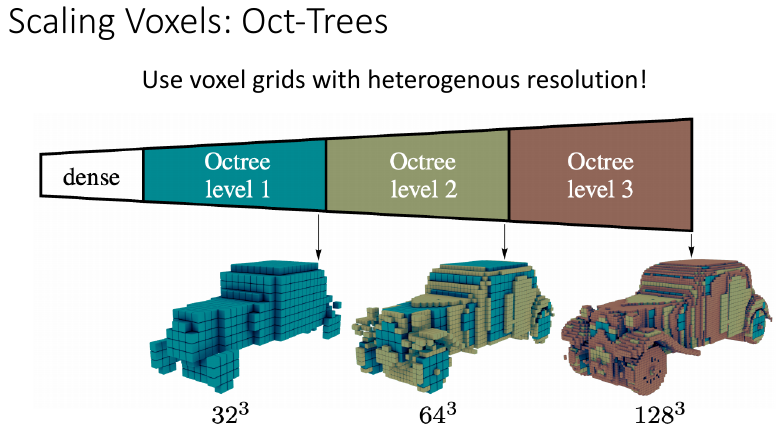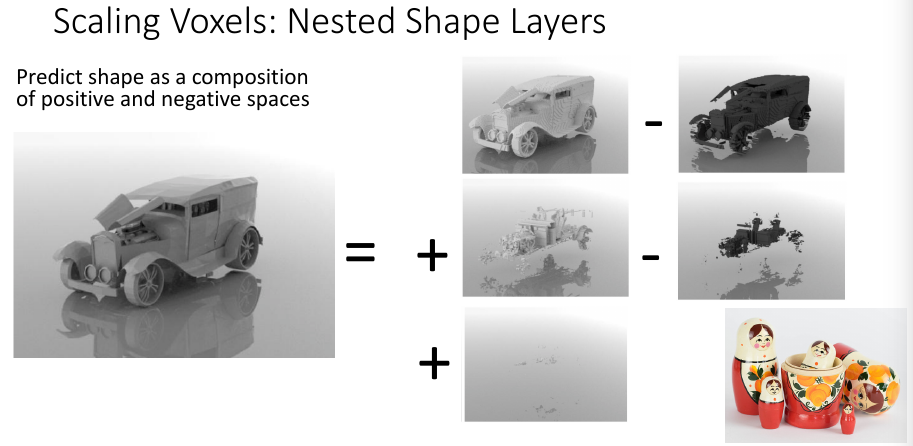### 2.3 隐式表面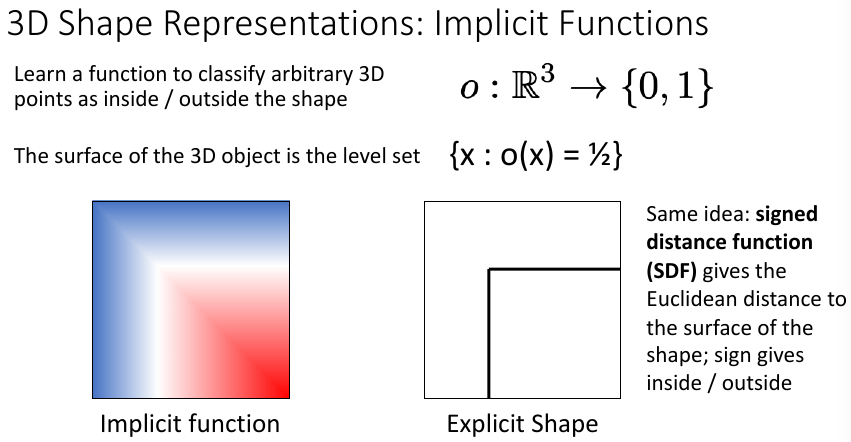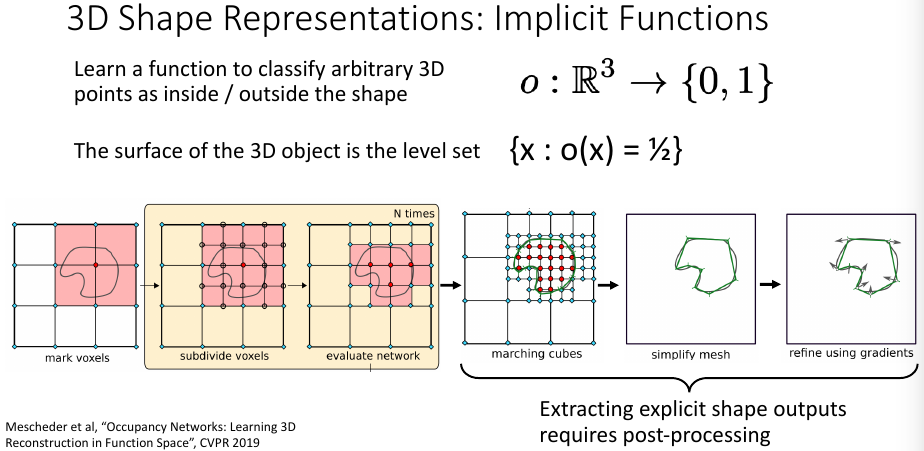### 2.4 点云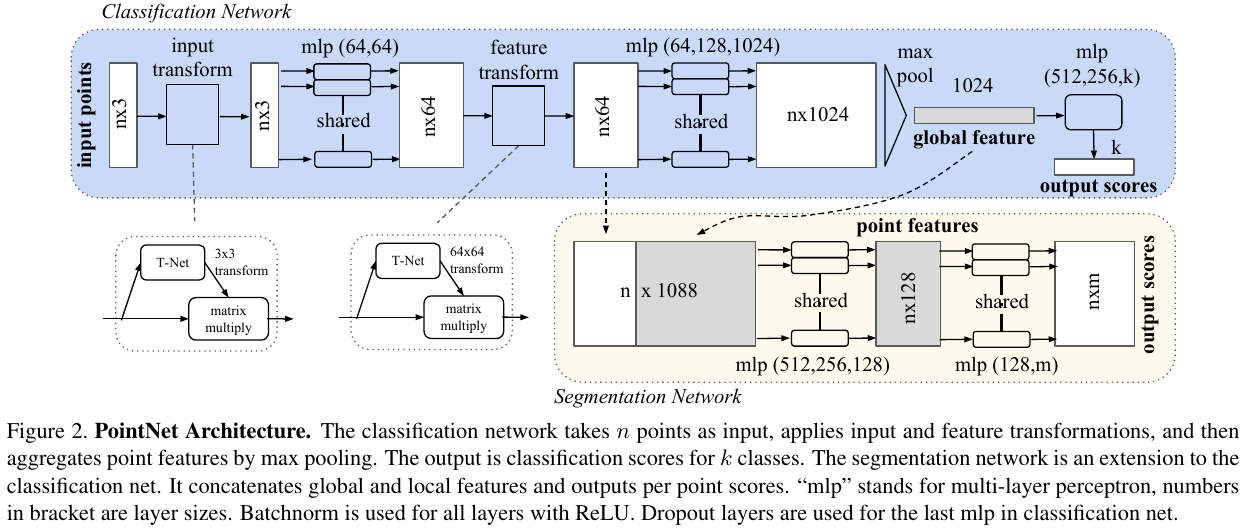PointNet 主要是通过使用 Max Pool 操作来消除数据顺序的影响。而要预测点云数据，也要消除数据顺序的影响。Fan 等人提出了 PointOutNet 来从 2D 图像预测得到 3D 点云数据：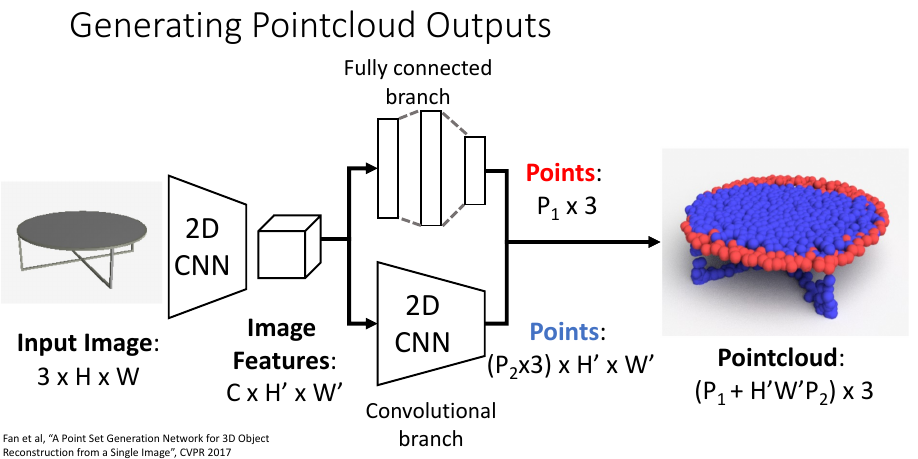• Chamfer Distance(CD)

$d_{\mathrm{CD}}(S_1, S_2) = \sum_{x \in S_1} \min_{y \in S_2} \lVert x - y \rVert_2^2 + \sum_{y \in S_2} \min_{x \in S_1} \lVert x - y \rVert_2^2 \tag{5}$

• Earth Mover's Distance(EMD)

$d_{\mathrm{EMD}}(S_1, S_2) = \min_{\phi: S_1 \rightarrow S_2} \sum_{x \in S_1} \lVert x - \phi(x) \rVert_2 \tag{6}$

其中，$\phi: S_1 \rightarrow S_2$ 是一个双射函数。

### 2.5 网格

• 顶点（Vertices）：3D 空间中的物体表面的点；
• 面（Faces）：顶点间的三角面。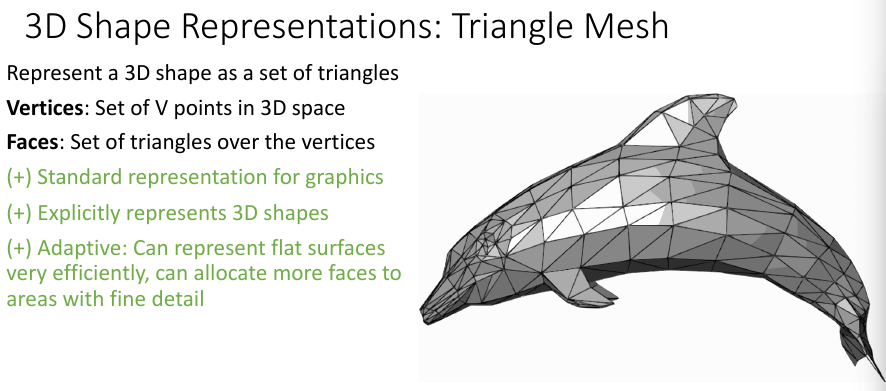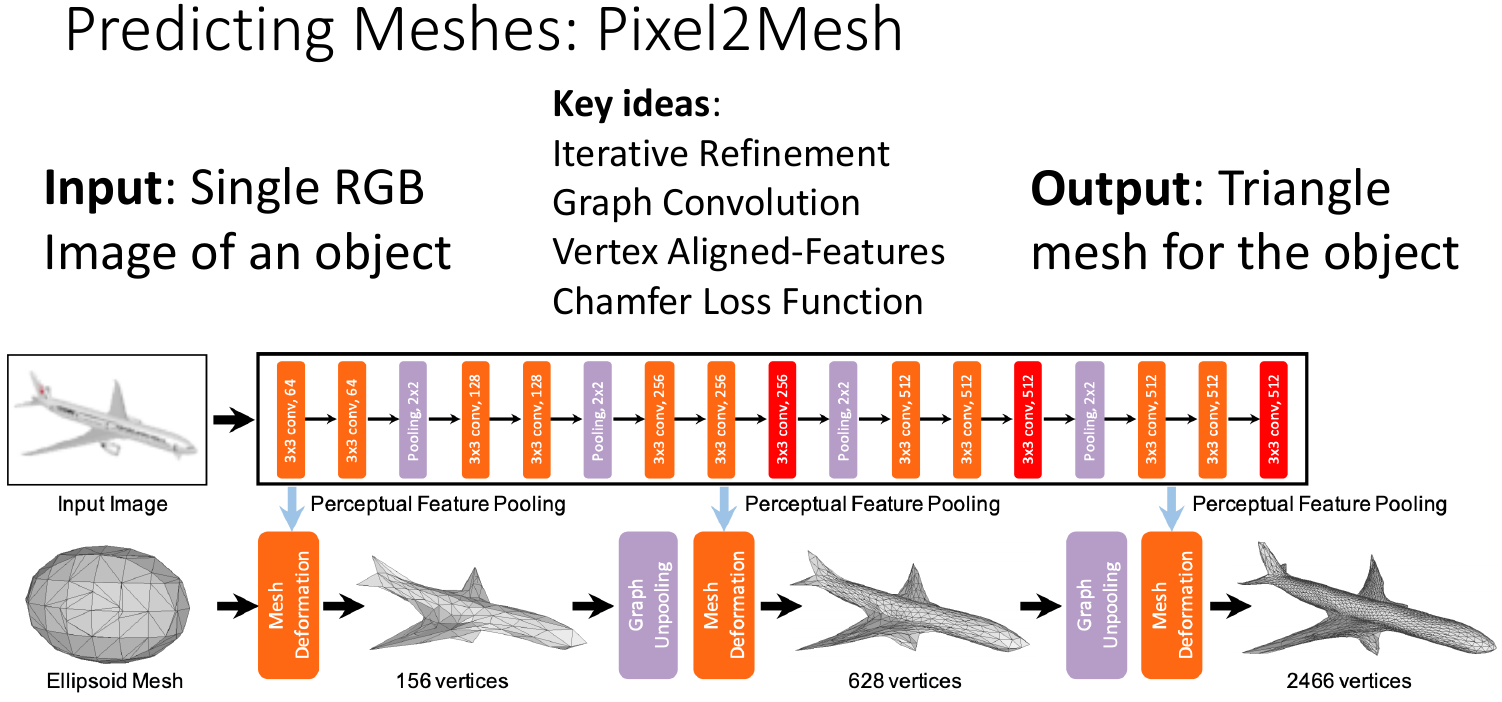Pixel2Mesh 的主要工作有两点：

• 迭代地精调：即捏面团的思想，增加顶点的方式是 Edge-based，而不是 Face-based。这是因为 Face-based 会导到顶点的度非常不平衡；

Edge-based 是在三角面的每条边中心增加一个点，然后连接这三个点，将一个大三角分成四个小三角，一次性增加了三个点；

Face-based 是指在三角面的中心新增一个点，然后将其和三个顶点连接，将一个大三角分成三个小三角，一次性增加一个点。

• 对齐顶点特征：为了和 2D 图像中物体的结构尽可能保持一致，可以投影或者插值的方式来对齐顶点特征。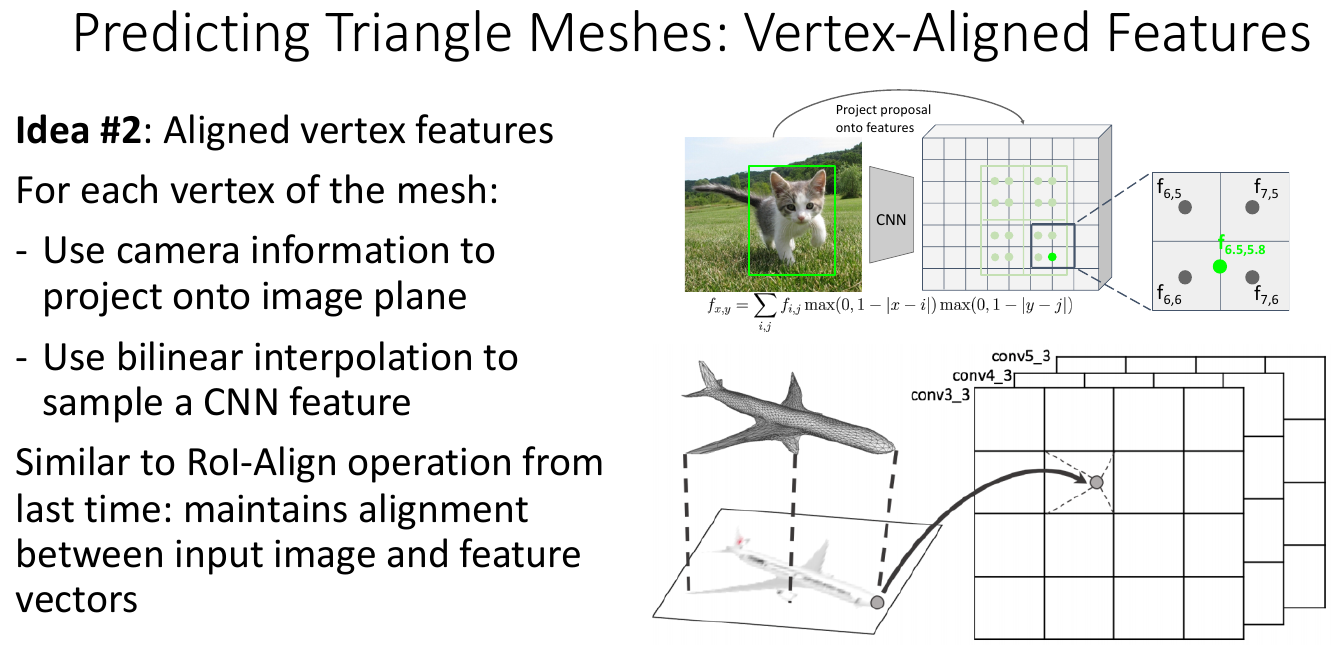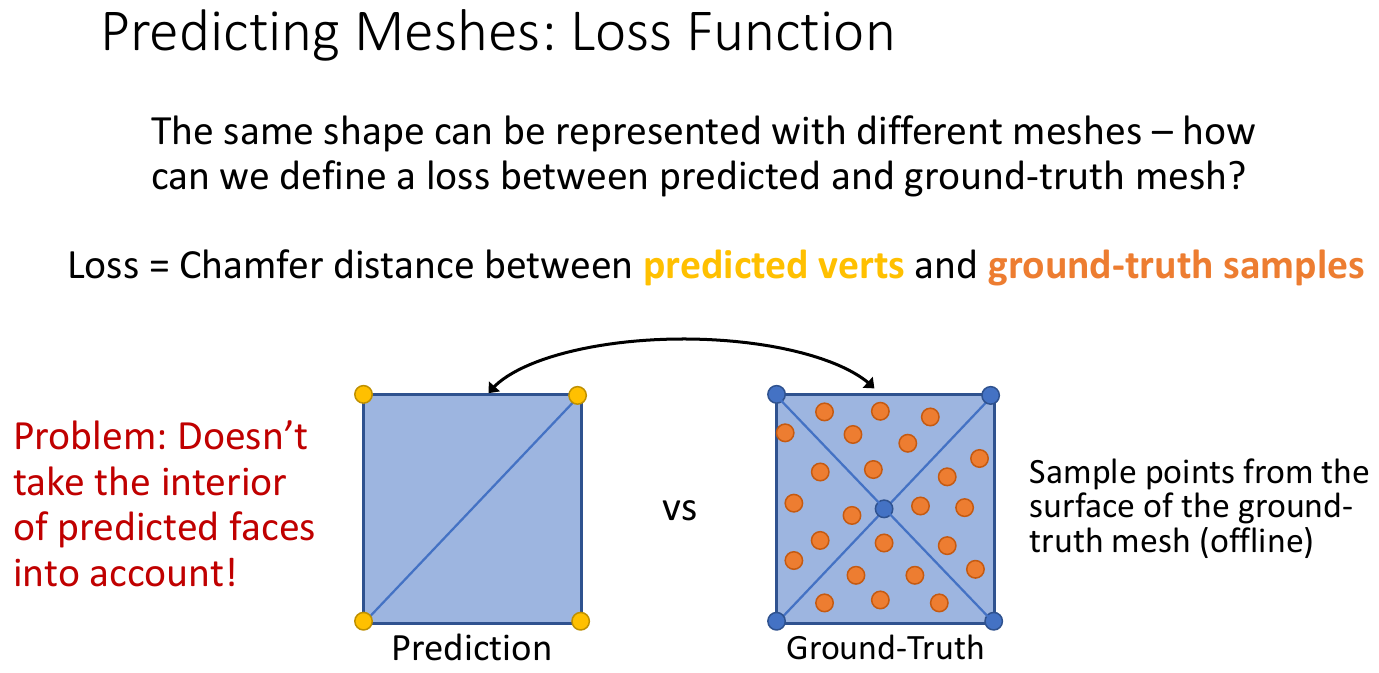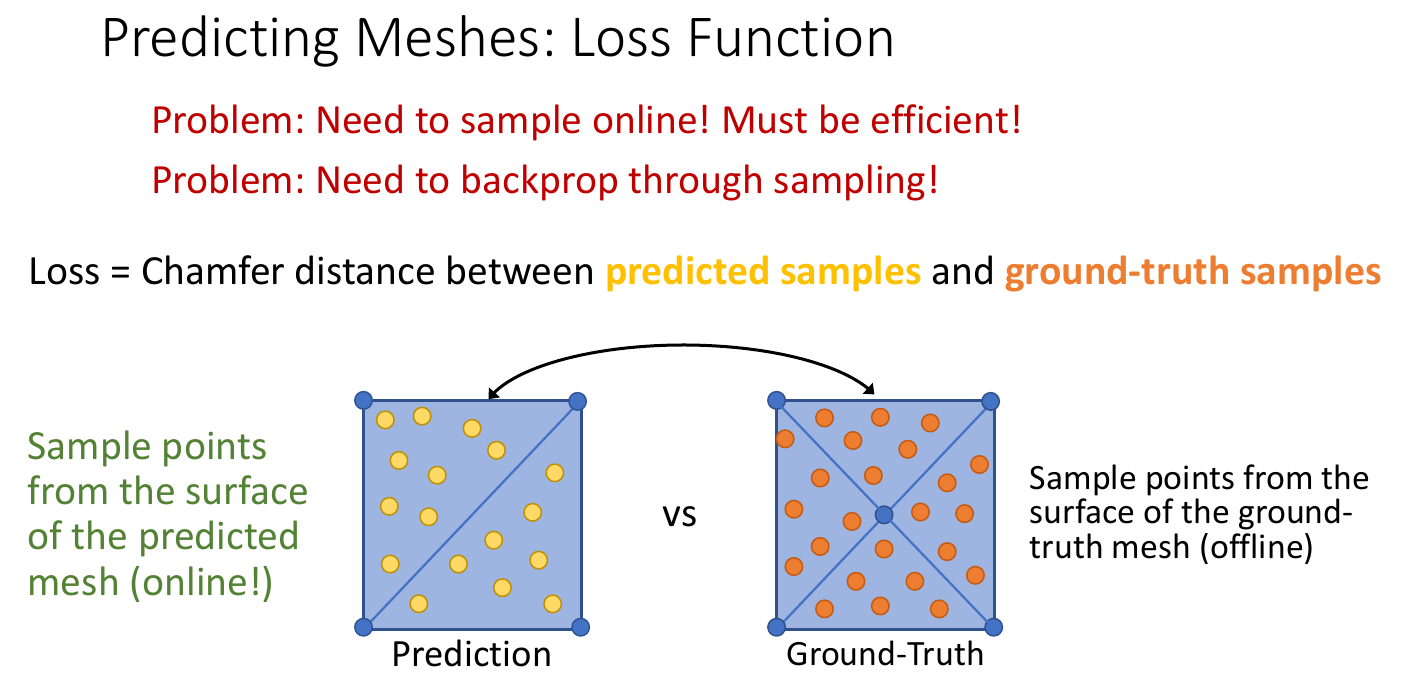## 3. 度量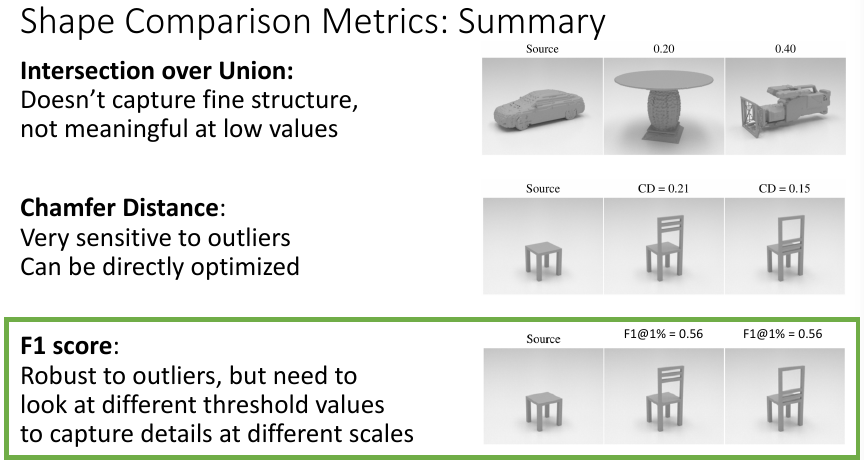### 3.1 IoU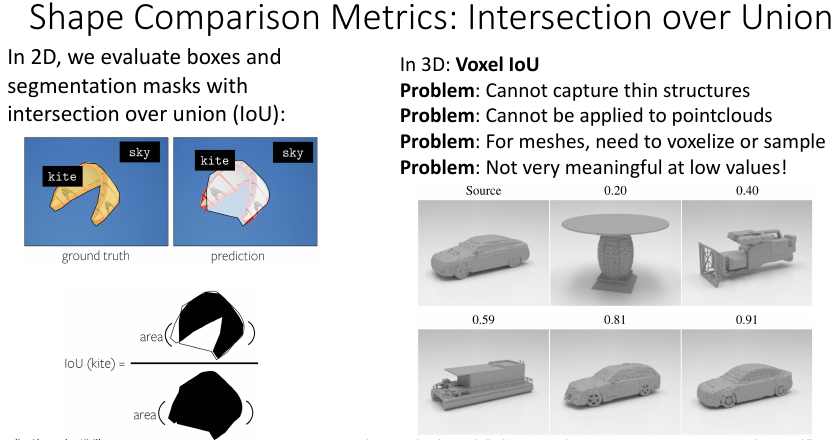### 3.2 Chamfer Distance

Chamfer Distance 前面也介绍了，本质是一种衡量集合间距离的度量函数。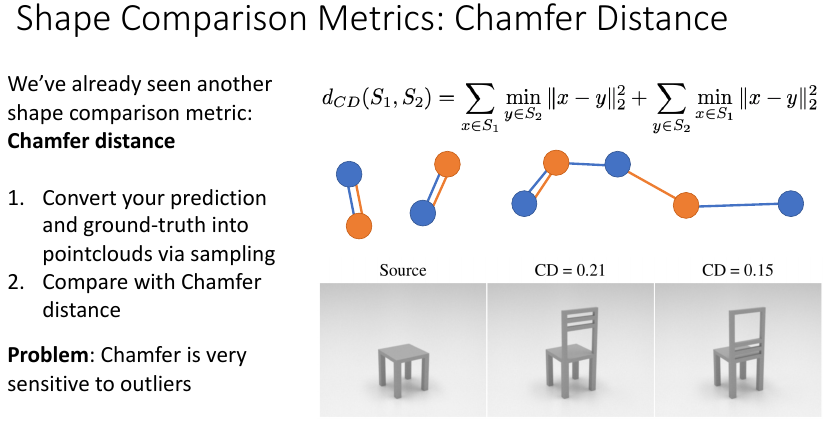### 3.3 F1 Score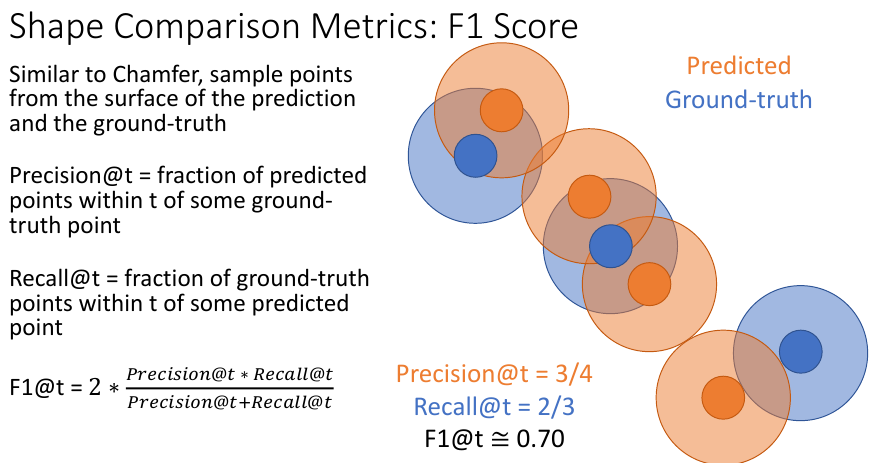## 4. 相机系统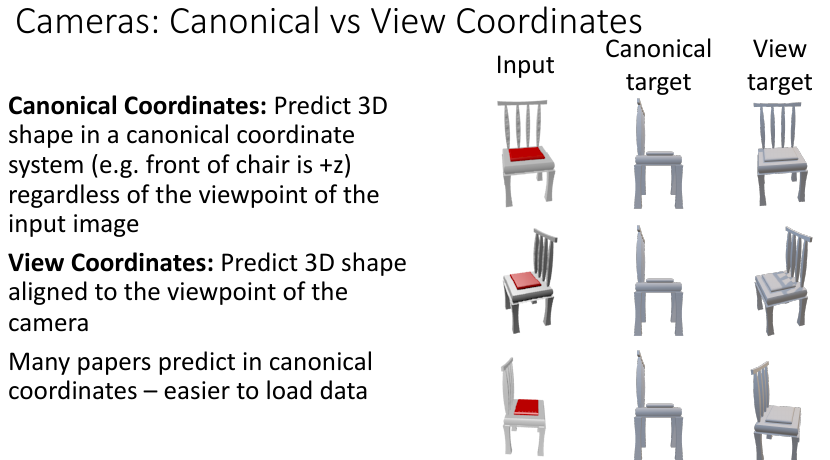### 4.1 Canonical Coordinates

Canonical Coordinates 系统以规范的方式来构建物体的坐标，比如上图所示，假定椅子的正前方为 $z$ 轴，然后依据右手法则构建出 $x-y-z$ 坐标系。这样做更容易加载数据，但不利于神经网络学习，因为此时神经网络永远只看到同一个视角。而且，由于这种方式，神经网络的输出也是规范视角下的，和原来的输入的视角不一致，因此还需要手动对齐。

### 4.2 View Coordinates

View Coordinates 则是以相机平面的法线为 $z$ 轴，这样则保留了输入的视角，也无需手动对齐输出，神经网络也能学到各种视角下的知识，模型也会更鲁棒。

## 5. 数据集

• ShapeNet：约 $50$ 个类，$50$k 个 3D CAD 模型，每个模型有 $25$ 张渲染图片（非真实数据集）；
• Pix3D$9$ 个类，$219$ 个 3D 模型，约 $17$k 张真实图像。

## 附录

### 参考文献

1. Eigen, D., Puhrsch, C., & Fergus, R. (2014). Depth map prediction from a single image using a multi-scale deep network. Advances in neural information processing systems, 27.
2. Eigen, D., & Fergus, R. (2015). Predicting depth, surface normals and semantic labels with a common multi-scale convolutional architecture. In Proceedings of the IEEE international conference on computer vision (pp. 2650-2658).
3. Wu, Z., Song, S., Khosla, A., Yu, F., Zhang, L., Tang, X., & Xiao, J. (2015). 3d shapenets: A deep representation for volumetric shapes. In Proceedings of the IEEE conference on computer vision and pattern recognition (pp. 1912-1920).
4. Choy, C. B., Xu, D., Gwak, J., Chen, K., & Savarese, S. (2016, October). 3d-r2n2: A unified approach for single and multi-view 3d object reconstruction. In European conference on computer vision (pp. 628-644). Springer, Cham.
5. Mescheder, L., Oechsle, M., Niemeyer, M., Nowozin, S., & Geiger, A. (2019). Occupancy networks: Learning 3d reconstruction in function space. In Proceedings of the IEEE/CVF Conference on Computer Vision and Pattern Recognition (pp. 4460-4470).
6. Qi, C. R., Su, H., Mo, K., & Guibas, L. J. (2017). Pointnet: Deep learning on point sets for 3d classification and segmentation. In Proceedings of the IEEE conference on computer vision and pattern recognition (pp. 652-660).
7. Qi, C. R., Yi, L., Su, H., & Guibas, L. J. (2017). Pointnet++: Deep hierarchical feature learning on point sets in a metric space. Advances in neural information processing systems, 30.
8. Fan, H., Su, H., & Guibas, L. J. (2017). A point set generation network for 3d object reconstruction from a single image. In Proceedings of the IEEE conference on computer vision and pattern recognition (pp. 605-613).
9. Wang, N., Zhang, Y., Li, Z., Fu, Y., Liu, W., & Jiang, Y. G. (2018). Pixel2mesh: Generating 3d mesh models from single rgb images. In Proceedings of the European conference on computer vision (ECCV) (pp. 52-67).
10. Smith, E. J., Fujimoto, S., Romero, A., & Meger, D. (2019). Geometrics: Exploiting geometric structure for graph-encoded objects. arXiv preprint arXiv:1901.11461.